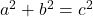Question

The sides of a right angled triangle has sides (x+1)cm, (x+2)cm and (x+4) cm.
i) Find the area of the triangle
ii) find the perimeter of the triangle
pls someone answer this. question ASAP. TNX ​

1.i)  (9 + 5√3)  cm²
ii)  (10 + 6√3)  cm
Step-by-step explanation:
Given sides of a right triangle:
• (x + 1) cm
• (x + 2) cm
• (x + 4) cm
The longest side of a right triangle is the hypotenuse.
The two shortest sides of a right triangle are the legs.
To find the value of x use Pythagoras Theorem.
Pythagoras Theoremwhere:
• a and b are the legs of the right triangle.
• c is the hypotenuse (longest side) of the right triangle.
Substitute the given expressions for the legs and hypotenuse into the formula:To find the value of x use the quadratic formula.Therefore:As  1 – 2√3 ≈ -2.46  we can discount this value of x as it would make two of the sides of the triangle negative values.  Since length cannot be negative, the only valid value of x is 1 + 2√3.
Therefore the lengths of the sides of the right triangle are:
• (1 + 2√3 + 1) = (2 + 2√3) cm
• (1 + 2√3 + 2) = (3 + 2√3) cm
• (1 + 2√3 + 4) = (5 + 2√3) cm

### Part (i)

Area of a trianglewhere:
• b = base
• h = height
The base and the height of a right triangle are its legs.
Substitute the found length of the legs into the formula and solve:### Part (ii)

The perimeter of a two-dimensional shape is the distance all the way around the outside. Therefore, the perimeter of a triangle is the sum of the lengths of its sides.2.diemkieu
Step-by-step explanation:
right-angled triangle.
that means Pythagoras applies :
c² = a² + b²
c being the Hypotenuse (the side opposite of the 90° angle and the longest of the 3 sides).
(x+4)² = (x+1)² + (x+2)²
x² + 8x + 16 = x² + 2x + 1 + x² + 4x + 4
8x + 16 = x² + 6x + 5
x² – 2x = 11
remember,
(x-a)² = x² -2ax + a²
compare to what we have
-2a = -2
a = 1
so, the full square on the left side is
(x-1)² = x² – 2x + 1
to complete the square in our equation we need to add 25 on both sides
x² – 2x + 1 = 11 + 1 = 12
(x – 1)² = 12
x – 1 = sqrt(12)
x = sqrt(12) + 1
and so
i)
when we have all 3 sides a, b, c, the area of the triangle is according to Heron’s formula
S = (a + b + c)/2
Area = sqrt(S(S-a)(S-b)(S-c))
in our case that is
S = ((x+1) + (x+2) + (x+4))/2 =
= ((sqrt(12)+1+1) + (sqrt(12)+1+2) + (sqrt(12)+1+4))
/2 = (3×sqrt(12) + 10)/2 =
= 10.19615242…
Area = sqrt(S(S- sqrt(12)-2)(S-sqrt(12)-3)(S-sqrt(12)-5)) =
= sqrt(10.19615242… ×
4.732050808… ×
3.732050808… ×
1.732050808…) =
= sqrt(311.8845727…) =
= 17.66025404… cm²
ii)
the perimeter is
(sqrt(12)+1+1) + (sqrt(12)+1+2) + (sqrt(12)+1+4) =
3sqrt(12) + 10 = 20.39230485… cm